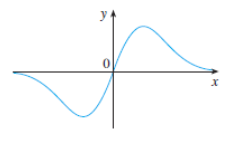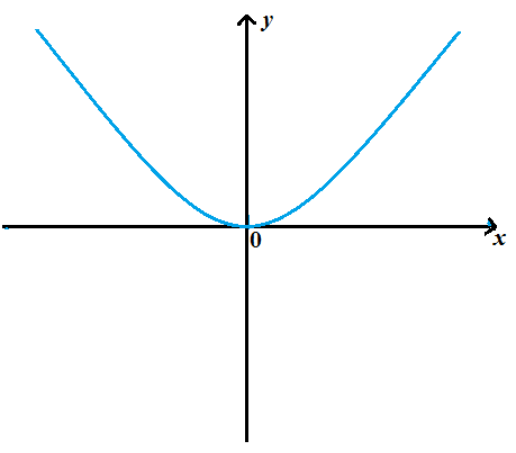# To graph: For the antiderivative of F , given that F ( 0 ) = 0 .### Single Variable Calculus: Concepts...

4th Edition
James Stewart
Publisher: Cengage Learning
ISBN: 9781337687805### Single Variable Calculus: Concepts...

4th Edition
James Stewart
Publisher: Cengage Learning
ISBN: 9781337687805

#### Solutions

Chapter 2, Problem 48RE
To determine

## To graph: For the antiderivative of F , given that F(0)=0 .

Expert Solution

### Explanation of Solution

Given information:

The graph of a function is shown in figure (1).Figure (1)

Graph:

From the given graph it is observed that for all negative values of x , the function lies below the x -axis. Which means F(x)<0 , for all x<0 .

This implies its anti-derivative function is decreasing in the interval (,0) .

For all positive values of x , the function lie above the x -axis, which means F(x)>0 , for all x>0 .

It means that its antiderivative function is increasing in the interval (0,) .

The graph for the antiderivative of the function is shown in figure (1).Figure (1)

Interpretation: Graph for theantiderivative of the function is shown in figure (1).

### Have a homework question?

Subscribe to bartleby learn! Ask subject matter experts 30 homework questions each month. Plus, you’ll have access to millions of step-by-step textbook answers!